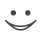# Get these flashcards, study & pass exams. For free! Even on iPhone/Android!

## ETAP 526- Lauren Steers (21 Cards)

What is the sin(30)?
1/2
What is the sin(45)?
sqrt(2)/2
What is the sin (60)?
sqrt(3)/2
What is the sin (90)?
1
What is the sin (180)?
0
What is the sin (270)
-1
What is the sin (0) or sin(360)?
0
What is the cos(0) or cos(360)?
1
What is the cos(30)?
sqrt(3)/2
What is the cos(45)?
sqrt(2)/2
What is the cos(60)?
1/2
What is the cos(90)?
0
What is the cos (180)?
-1
What is the cos (270)?
0
What is the tan (0) or tan (360)?
0
What is the tan (30)?
1/sqrt(3) = sqrt(3)/3
What is the tan (45)?
1
What is the tan (60)?
sqrt(3)
What is the tan (90)?
undefined
What is the tan (180)?
0
What is the tan (270)?
undefinedFlashcard set info:
Author: lsteers10
Main topic: Mathematics
Topic: Trigonometry
School / Univ.: SUNY Albany
City: Albany
Published: 06.04.2014
Tags: Teacher

Card tags:
All cards (21)
no tagsReport abuse